# RD Sharma Solutions for Class 11 Maths Exercise 14.1 Chapter 14 – Quadratic Equations

In Exercise 14.1 of Chapter 14, we shall study some definitions and discuss problems based on quadratic equations with real coefficients. To help improve conceptual knowledge among students, experts have designed the solutions, as per CBSE guidelines. The PDF of RD Sharma Class 11 Solutions is provided here. Students are advised to practice the solutions on a daily basis to achieve their goals of scoring high marks in their board exams. Learners can easily access and download the solutions as their study material.

## Download the pdf of RD Sharma Solutions for Class 11 Maths Exercise 14.1 Chapter 14 – Quadratic Equations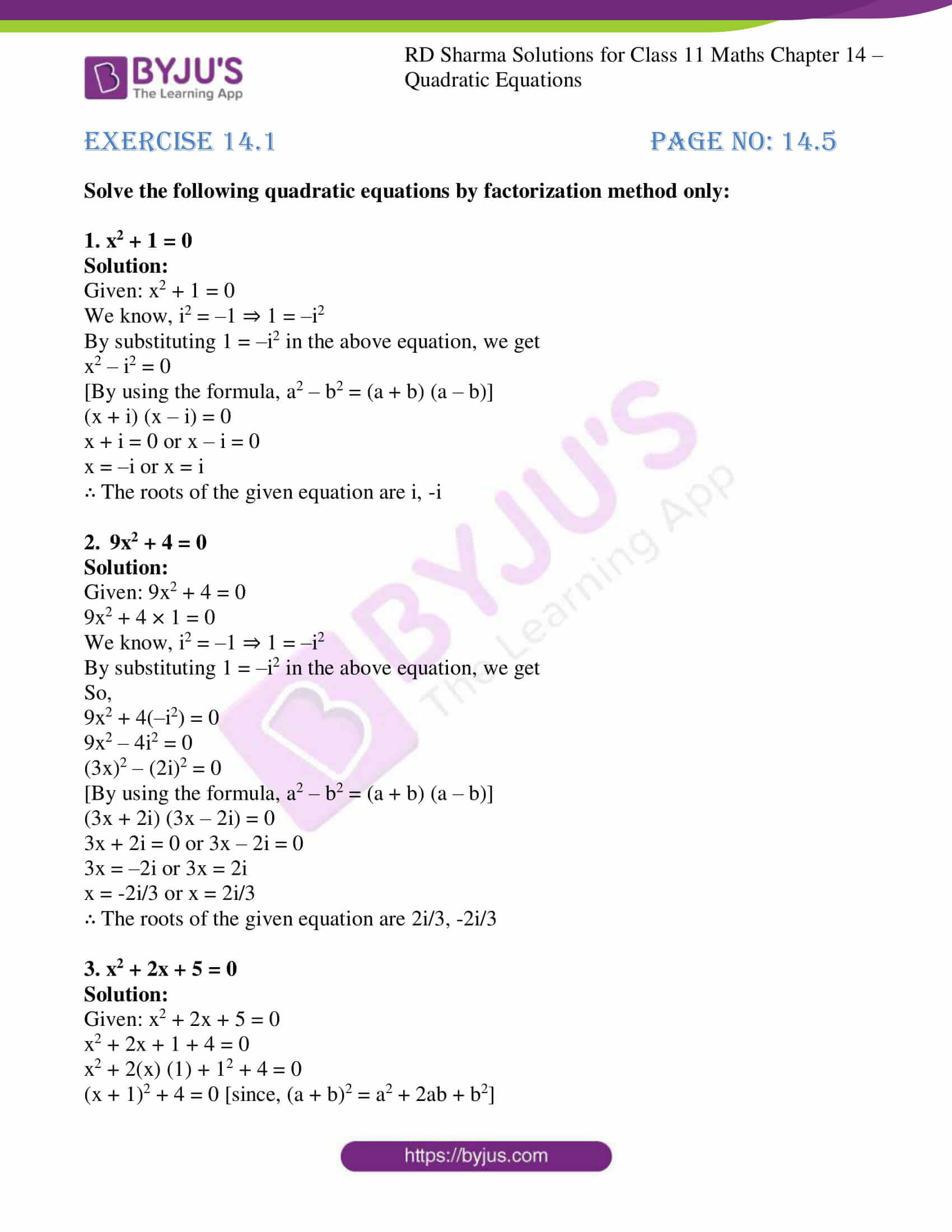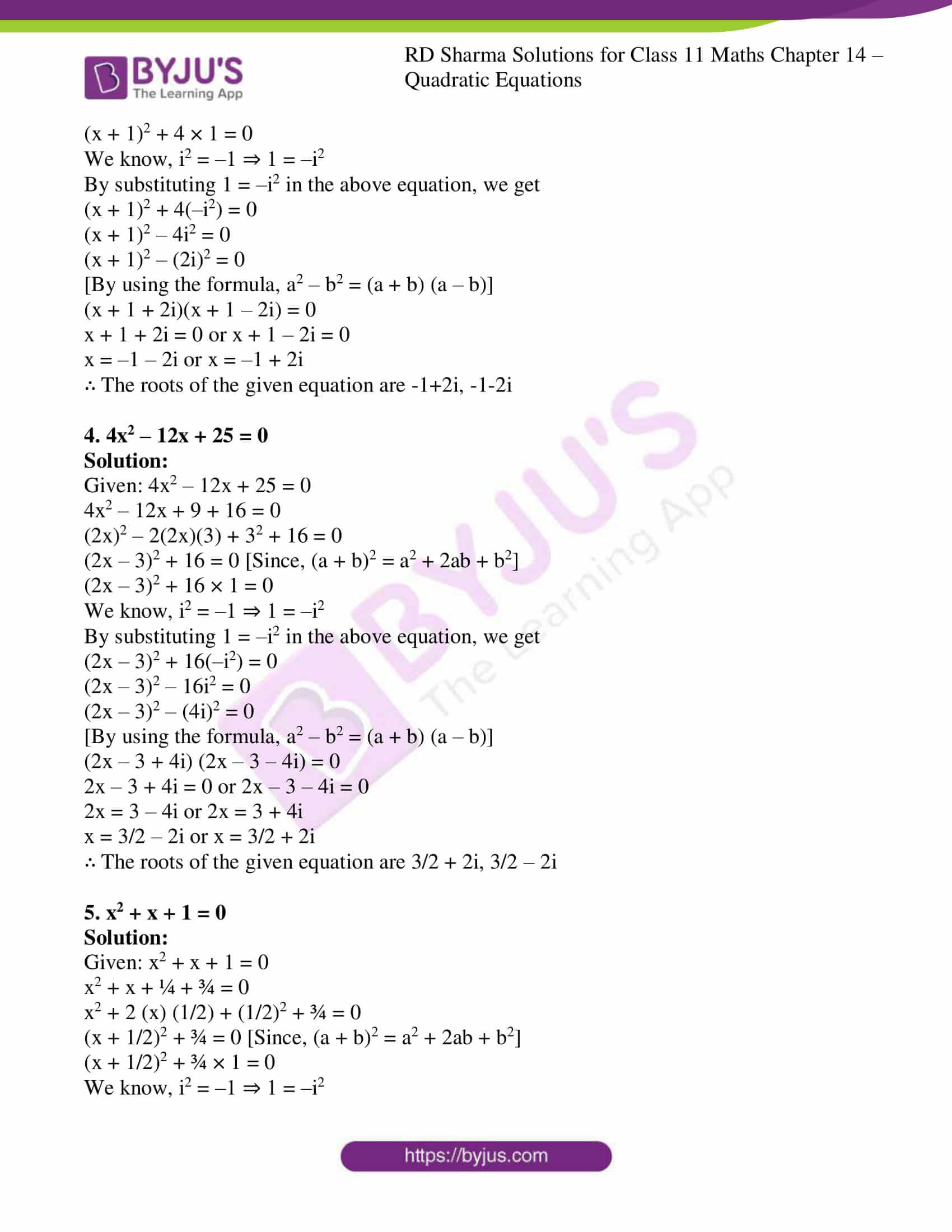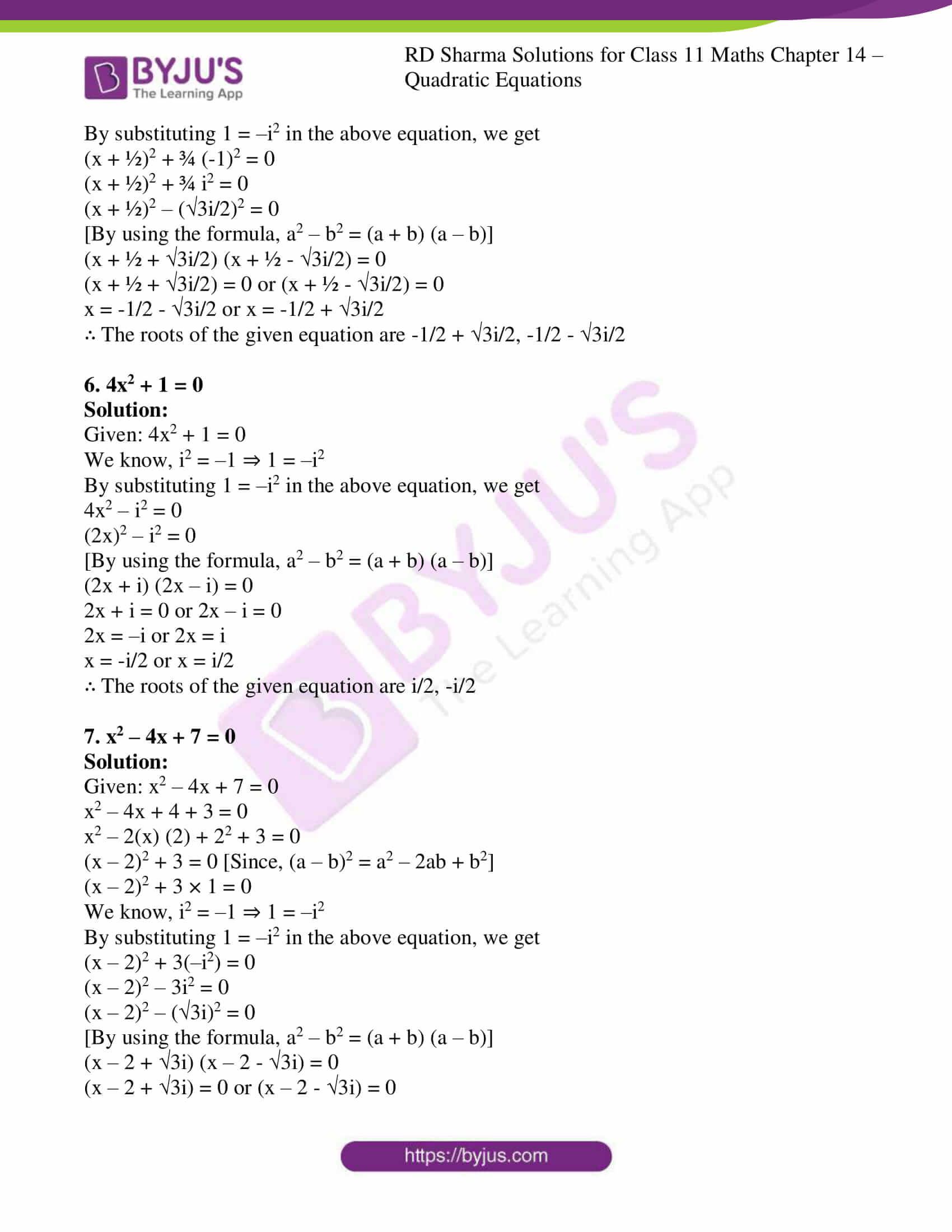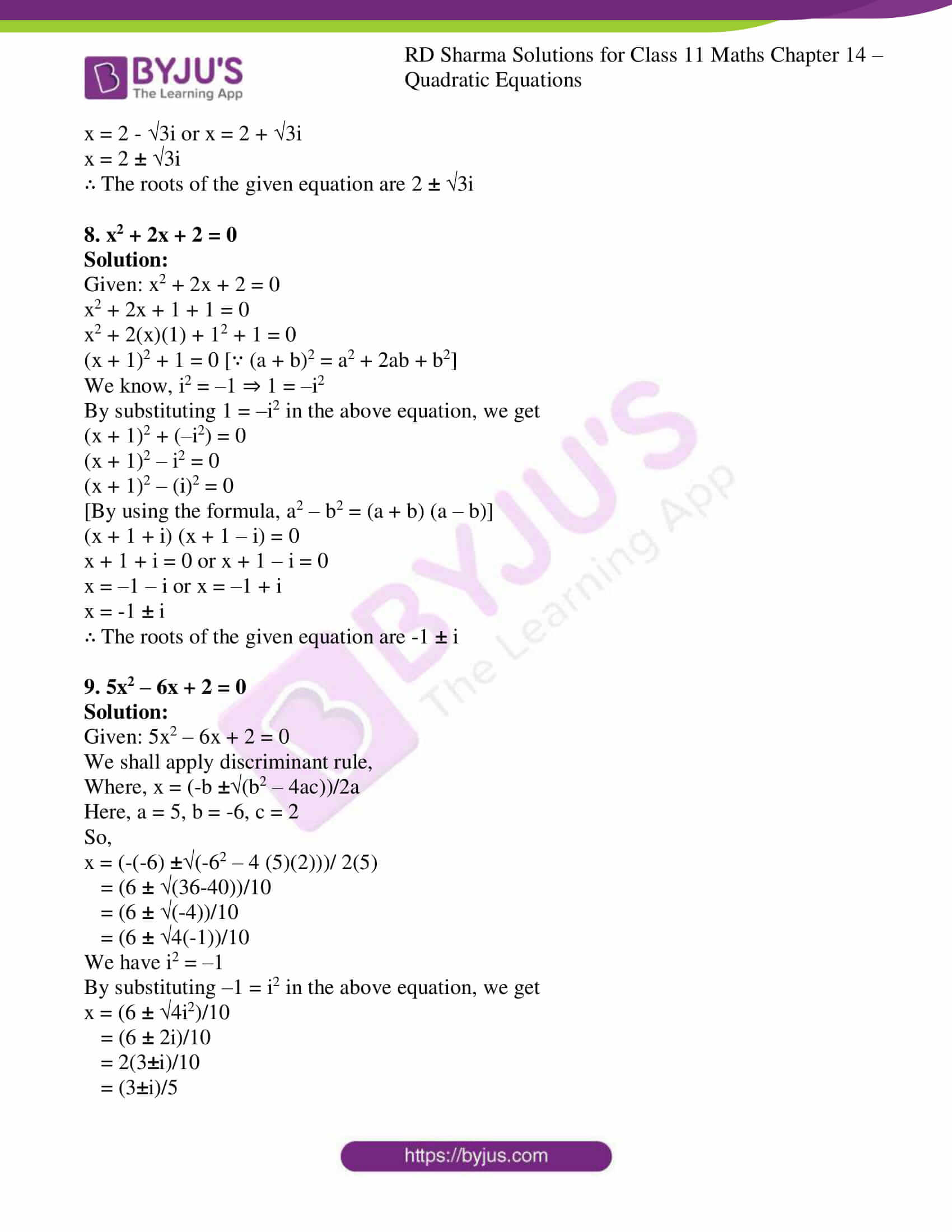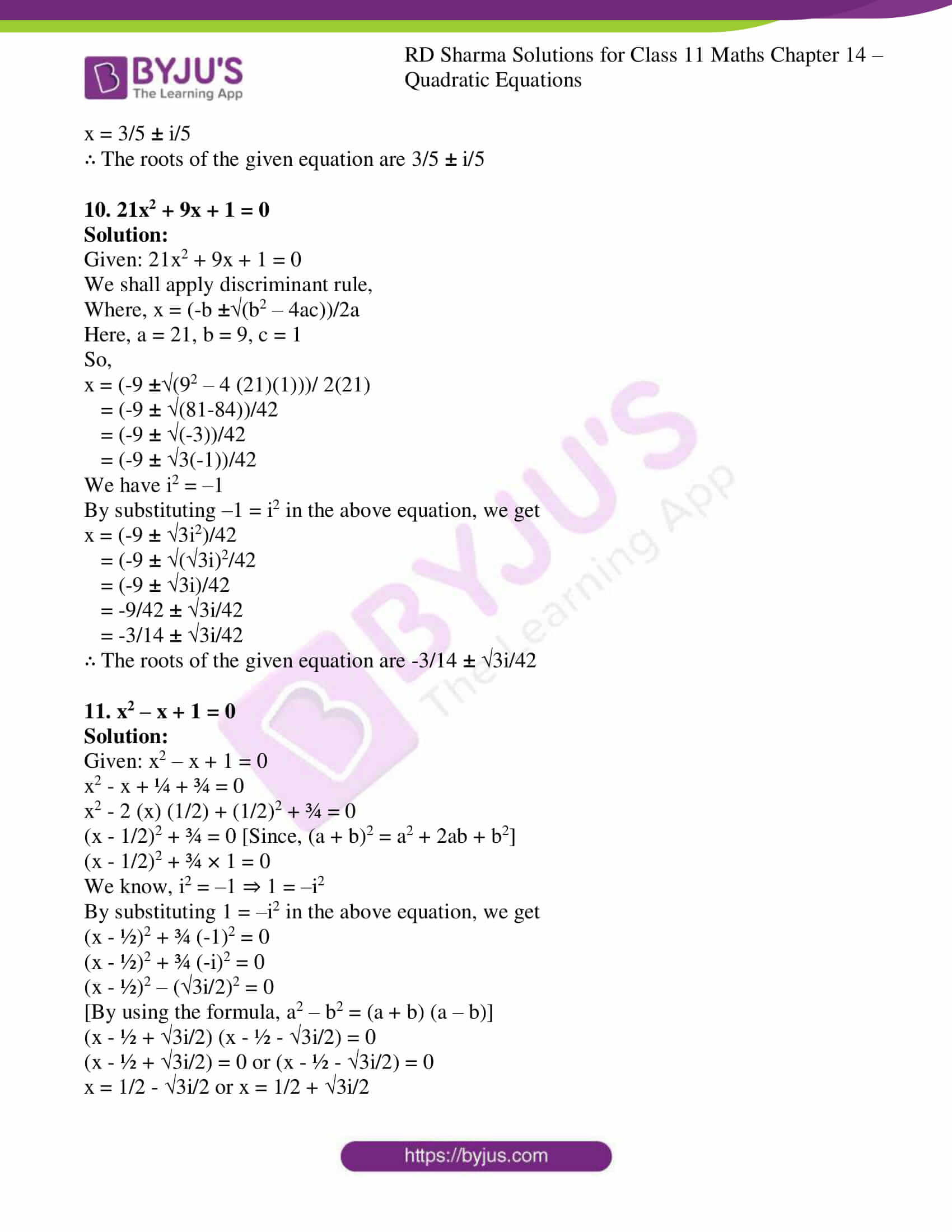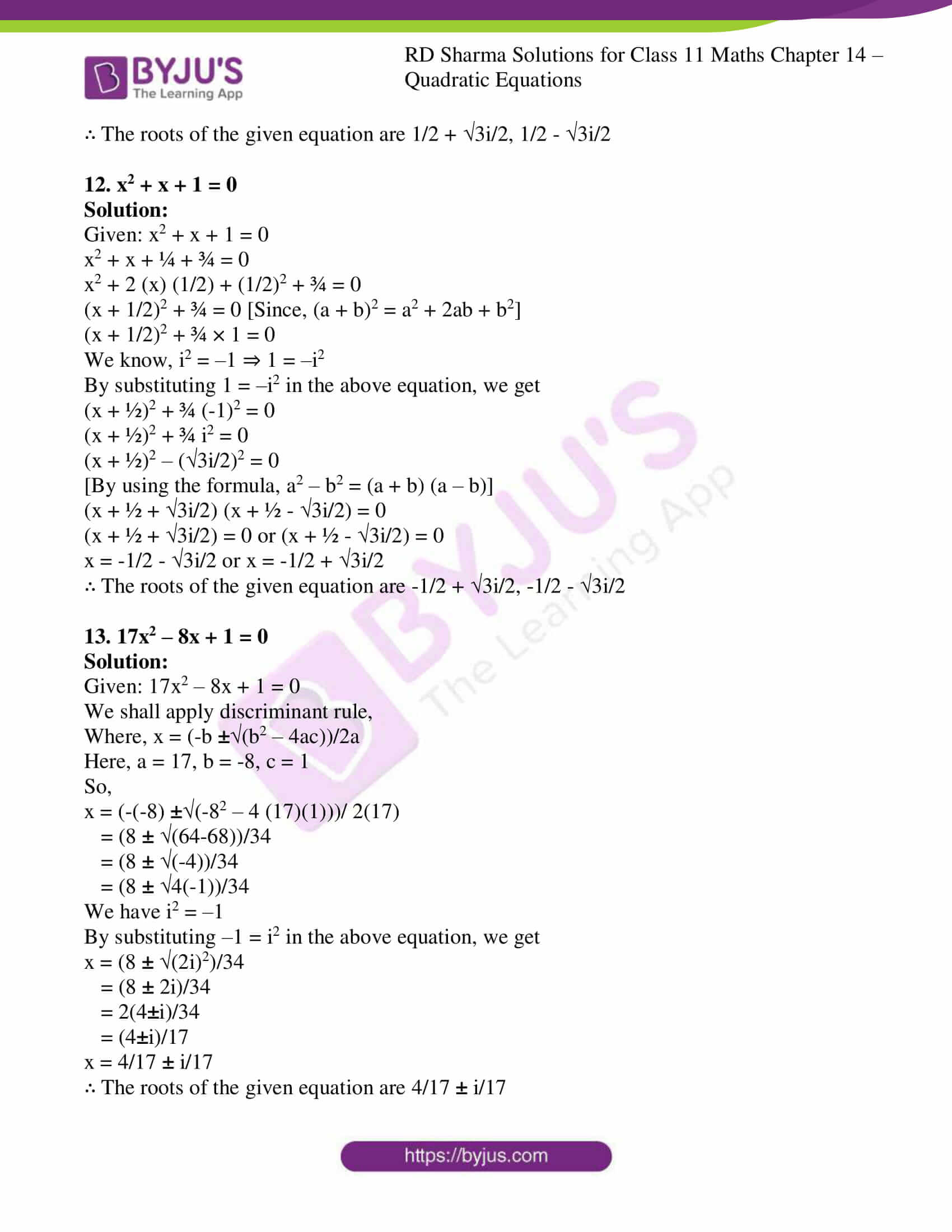### Also, access another exercise of RD Sharma Solutions for Class 11 Maths Chapter 14 – Quadratic Equations

Exercise 14.2 Solutions

### Access answers to RD Sharma Solutions for Class 11 Maths Exercise 14.1 Chapter 14 – Quadratic Equations

Solve the following quadratic equations by factorization method only:

1. x2 + 1 = 0

Solution:

Given: x2 + 1 = 0

We know, i2 = –1 ⇒ 1 = –i2

By substituting 1 = –i2 in the above equation, we get

x2 – i2 = 0

[By using the formula, a2 – b2 = (a + b) (a – b)]

(x + i) (x – i) = 0

x + i = 0 or x – i = 0

x = –i or x = i

∴ The roots of the given equation are i, -i

2. 9x2 + 4 = 0

Solution:

Given: 9x2 + 4 = 0

9x2 + 4 × 1 = 0

We know, i2 = –1 ⇒ 1 = –i2

By substituting 1 = –i2 in the above equation, we get

So,

9x2 + 4(–i2) = 0

9x2 – 4i2 = 0

(3x)2 – (2i)2 = 0

[By using the formula, a2 – b2 = (a + b) (a – b)]

(3x + 2i) (3x – 2i) = 0

3x + 2i = 0 or 3x – 2i = 0

3x = –2i or 3x = 2i

x = -2i/3 or x = 2i/3

∴ The roots of the given equation are 2i/3, -2i/3

3. x2 + 2x + 5 = 0

Solution:

Given: x2 + 2x + 5 = 0

x2 + 2x + 1 + 4 = 0

x2 + 2(x) (1) + 12 + 4 = 0

(x + 1)2 + 4 = 0 [since, (a + b)2 = a2 + 2ab + b2]

(x + 1)2 + 4 × 1 = 0

We know, i2 = –1 ⇒ 1 = –i2

By substituting 1 = –i2 in the above equation, we get

(x + 1)2 + 4(–i2) = 0

(x + 1)2 – 4i2 = 0

(x + 1)2 – (2i)2 = 0

[By using the formula, a2 – b2 = (a + b) (a – b)]

(x + 1 + 2i)(x + 1 – 2i) = 0

x + 1 + 2i = 0 or x + 1 – 2i = 0

x = –1 – 2i or x = –1 + 2i

∴ The roots of the given equation are -1+2i, -1-2i

4. 4x2 – 12x + 25 = 0

Solution:

Given: 4x2 – 12x + 25 = 0

4x2 – 12x + 9 + 16 = 0

(2x)2 – 2(2x)(3) + 32 + 16 = 0

(2x – 3)2 + 16 = 0 [Since, (a + b)2 = a2 + 2ab + b2]

(2x – 3)2 + 16 × 1 = 0

We know, i2 = –1 ⇒ 1 = –i2

By substituting 1 = –i2 in the above equation, we get

(2x – 3)2 + 16(–i2) = 0

(2x – 3)2 – 16i2 = 0

(2x – 3)2 – (4i)2 = 0

[By using the formula, a2 – b2 = (a + b) (a – b)]

(2x – 3 + 4i) (2x – 3 – 4i) = 0

2x – 3 + 4i = 0 or 2x – 3 – 4i = 0

2x = 3 – 4i or 2x = 3 + 4i

x = 3/2 – 2i or x = 3/2 + 2i

∴ The roots of the given equation are 3/2 + 2i, 3/2 – 2i

5. x2 + x + 1 = 0

Solution:

Given: x2 + x + 1 = 0

x2 + x + ¼ + ¾ = 0

x2 + 2 (x) (1/2) + (1/2)2 + ¾ = 0

(x + 1/2)2 + ¾ = 0 [Since, (a + b)2 = a2 + 2ab + b2]

(x + 1/2)2 + ¾ × 1 = 0

We know, i2 = –1 ⇒ 1 = –i2

By substituting 1 = –i2 in the above equation, we get

(x + ½)2 + ¾ (-1)2 = 0

(x + ½)2 + ¾ i2 = 0

(x + ½)2 – (3i/2)2 = 0

[By using the formula, a2 – b2 = (a + b) (a – b)]

(x + ½ + 3i/2) (x + ½ – 3i/2) = 0

(x + ½ + 3i/2) = 0 or (x + ½ – 3i/2) = 0

x = -1/2 – 3i/2 or x = -1/2 + 3i/2

∴ The roots of the given equation are -1/2 + 3i/2, -1/2 – 3i/2

6. 4x2 + 1 = 0

Solution:

Given: 4x2 + 1 = 0

We know, i2 = –1 ⇒ 1 = –i2

By substituting 1 = –i2 in the above equation, we get

4x2 – i2 = 0

(2x)2 – i2 = 0

[By using the formula, a2 – b2 = (a + b) (a – b)]

(2x + i) (2x – i) = 0

2x + i = 0 or 2x – i = 0

2x = –i or 2x = i

x = -i/2 or x = i/2

∴ The roots of the given equation are i/2, -i/2

7. x2 – 4x + 7 = 0

Solution:

Given: x2 – 4x + 7 = 0

x2 – 4x + 4 + 3 = 0

x2 – 2(x) (2) + 22 + 3 = 0

(x – 2)2 + 3 = 0 [Since, (a – b)2 = a2 – 2ab + b2]

(x – 2)2 + 3 × 1 = 0

We know, i2 = –1 ⇒ 1 = –i2

By substituting 1 = –i2 in the above equation, we get

(x – 2)2 + 3(–i2) = 0

(x – 2)2 – 3i2 = 0

(x – 2)2 – (3i)2 = 0

[By using the formula, a2 – b2 = (a + b) (a – b)]

(x – 2 + 3i) (x – 2 – 3i) = 0

(x – 2 + 3i) = 0 or (x – 2 – 3i) = 0

x = 2 – 3i or x = 2 + 3i

x = 2 ± 3i

∴ The roots of the given equation are 2 ± 3i

8. x2 + 2x + 2 = 0

Solution:

Given: x2 + 2x + 2 = 0

x2 + 2x + 1 + 1 = 0

x2 + 2(x)(1) + 12 + 1 = 0

(x + 1)2 + 1 = 0 [∵ (a + b)2 = a2 + 2ab + b2]

We know, i2 = –1 ⇒ 1 = –i2

By substituting 1 = –i2 in the above equation, we get

(x + 1)2 + (–i2) = 0

(x + 1)2 – i2 = 0

(x + 1)2 – (i)2 = 0

[By using the formula, a2 – b2 = (a + b) (a – b)]

(x + 1 + i) (x + 1 – i) = 0

x + 1 + i = 0 or x + 1 – i = 0

x = –1 – i or x = –1 + i

x = -1 ± i

∴ The roots of the given equation are -1 ± i

9. 5x2 – 6x + 2 = 0

Solution:

Given: 5x2 – 6x + 2 = 0

We shall apply discriminant rule,

Where, x = (-b ±√(b2 – 4ac))/2a

Here, a = 5, b = -6, c = 2

So,

x = (-(-6) ±√(-62 – 4 (5)(2)))/ 2(5)

= (6 ± √(36-40))/10

= (6 ± √(-4))/10

= (6 ± √4(-1))/10

We have i2 = –1

By substituting –1 = i2 in the above equation, we get

x = (6 ± √4i2)/10

= (6 ± 2i)/10

= 2(3±i)/10

= (3±i)/5

x = 3/5 ± i/5

∴ The roots of the given equation are 3/5 ± i/5

10. 21x2 + 9x + 1 = 0

Solution:

Given: 21x2 + 9x + 1 = 0

We shall apply discriminant rule,

Where, x = (-b ±√(b2 – 4ac))/2a

Here, a = 21, b = 9, c = 1

So,

x = (-9 ±√(92 – 4 (21)(1)))/ 2(21)

= (-9 ± √(81-84))/42

= (-9 ± √(-3))/42

= (-9 ± √3(-1))/42

We have i2 = –1

By substituting –1 = i2 in the above equation, we get

x = (-9 ± √3i2)/42

= (-9 ± √(3i)2/42

= (-9 ± √3i)/42

= -9/42 ± √3i/42

= -3/14 ± √3i/42

∴ The roots of the given equation are -3/14 ± √3i/42

11. x2 – x + 1 = 0

Solution:

Given: x2 – x + 1 = 0

x2 – x + ¼ + ¾ = 0

x2 – 2 (x) (1/2) + (1/2)2 + ¾ = 0

(x – 1/2)2 + ¾ = 0 [Since, (a + b)2 = a2 + 2ab + b2]

(x – 1/2)2 + ¾ × 1 = 0

We know, i2 = –1 ⇒ 1 = –i2

By substituting 1 = –i2 in the above equation, we get

(x – ½)2 + ¾ (-1)2 = 0

(x – ½)2 + ¾ (-i)2 = 0

(x – ½)2 – (3i/2)2 = 0

[By using the formula, a2 – b2 = (a + b) (a – b)]

(x – ½ + 3i/2) (x – ½ – 3i/2) = 0

(x – ½ + 3i/2) = 0 or (x – ½ – 3i/2) = 0

x = 1/2 – 3i/2 or x = 1/2 + 3i/2

∴ The roots of the given equation are 1/2 + 3i/2, 1/2 – 3i/2

12. x2 + x + 1 = 0

Solution:

Given: x2 + x + 1 = 0

x2 + x + ¼ + ¾ = 0

x2 + 2 (x) (1/2) + (1/2)2 + ¾ = 0

(x + 1/2)2 + ¾ = 0 [Since, (a + b)2 = a2 + 2ab + b2]

(x + 1/2)2 + ¾ × 1 = 0

We know, i2 = –1 ⇒ 1 = –i2

By substituting 1 = –i2 in the above equation, we get

(x + ½)2 + ¾ (-1)2 = 0

(x + ½)2 + ¾ i2 = 0

(x + ½)2 – (3i/2)2 = 0

[By using the formula, a2 – b2 = (a + b) (a – b)]

(x + ½ + 3i/2) (x + ½ – 3i/2) = 0

(x + ½ + 3i/2) = 0 or (x + ½ – 3i/2) = 0

x = -1/2 – 3i/2 or x = -1/2 + 3i/2

∴ The roots of the given equation are -1/2 + 3i/2, -1/2 – 3i/2

13. 17x2 – 8x + 1 = 0

Solution:

Given: 17x2 – 8x + 1 = 0

We shall apply discriminant rule,

Where, x = (-b ±√(b2 – 4ac))/2a

Here, a = 17, b = -8, c = 1

So,

x = (-(-8) ±√(-82 – 4 (17)(1)))/ 2(17)

= (8 ± √(64-68))/34

= (8 ± √(-4))/34

= (8 ± √4(-1))/34

We have i2 = –1

By substituting –1 = i2 in the above equation, we get

x = (8 ± √(2i)2)/34

= (8 ± 2i)/34

= 2(4±i)/34

= (4±i)/17

x = 4/17 ± i/17

∴ The roots of the given equation are 4/17 ± i/17